# 摘要

1. 简要介绍数字图像处理涉及的一些基本概念、基本运算、基本类型，以及如何通过Python对数字图像进行读取和简单操作。
2. 以彩色图像为例对数字图像处理的基本操作进行介绍，熟悉数字图像处理的基本过程，主要包括颜色空间的基本概念、伪彩色图像处理操作，彩色图像处理简单操作。
3. 瞄准在空间域中对图像进行增强，介绍空间滤波的机理、基本概念以及使用的基本技术。本章内容包括空间滤波基本概念、基于空间滤波的图像平滑处理、基于空间滤波的锐化操作以及混合空间增强。
4. 从频域角度入手对图像处理及增强方法展开介绍。因为频域滤波所需的数学知识较多，所以本章采取由浅入深的策略，首先介绍一维傅里叶变换，其次介绍二维傅里叶变换和快速傅里叶变换，最后介绍图像频域滤波中出现的各种技术，其大体可分为低通滤波和高通滤波两大类。
5. 从全局特征提取和局部特征提取两方面入手，分别介绍颜色特征、纹理特征、形状特征、边缘特征、点特征的提取方法。本章内容是目前机器视觉和图像处理领域的学者关注较多的内容，通过穿插较多的实例，帮助读者理解图像特征提取的基本技术。
6. 瞄准如何减少图像传输及存储数据大小，介绍主要使用的压缩技术，包括有损压缩和无损压缩等，并使用`JPEG`压缩技术串讲全章知识点。
7. 介绍图像的小波域表示及多分辨率表示。

# 绪论

• 人工智能是引领未来发展的战略性技术，是新一轮科技革命和产业变革的重要驱动力量，将深刻地改变人类社会生活。

• 促进人工智能和实体经济的深度融合，构建数据驱动、人机协同、跨界融合、共创分享的智能经济形态，更是推动质量变革、效率变革、动力变革的重要途经。

• 进年来，我国人工智能新技术、新产品、新业态持续涌现，与农业、制造业、服务业等行业的融合步伐明显加快，在技术创新、应用推广、产业发展等方面成效初显。

• 人工智能技术并不是一个新生事物，它在最近几年引起全球性关注并得到飞速发展的主要原因，在于它的三个基本要素（算法、数据、算力）的迅猛发展，其中又以数据和算力的发展尤为重要。

• 物联网技术的蓬勃发展使得数据累计的难度越来越低，而芯片算力的不断提升，使得过去只能通过云计算才能完成的人工智能运算，现在可以下沉到最普通的设备上完成。

• 物联网技术为机器带来感知能力，而人工智能则通过计算算力为机器带来了决策能力，正如感知和大脑对自然生命进化所起到的必然性作用。

# 1 数字图像处理基础知识

https://hulin.blog.csdn.net/article/details/107570020

# 2 彩色图像处理初步

https://hulin.blog.csdn.net/article/details/107578369

# 3 空间滤波

https://hulin.blog.csdn.net/article/details/107589248

# 4 频域滤波

https://hulin.blog.csdn.net/article/details/107609844

# 5 图像特征提取

• 图像特征是指可以对图像的内容或特点，进行表征的一系列数学的集合，主要包括图像的自然特征（如亮度、色彩、纹理等）和图像人为特征（如图像频谱、图像直方图等）。

• 图像特征提取可以视为广义上的图像变换，即将图像从原始属性空间转化到特征属性空间。

• 图像特征提取过程是指对图像包含的信息进行处理和分析，并将其中不易受随机因素干扰的信息，作为图像的特征提取出来，进而实现将图像的原始特征，表示为一组具有明显的物理意义或统计意义的特征。

• 图像特征提取之后，通常还会伴随图像特征的选择。

• 图像特征选择过程是去除冗余信息的过程，其具有提高识别精度、减少运算量、提高运算速度等作用。

• 良好的图像特征通常具有以下3个特征。
① 代表性或可区分性
图像特征应能够对该类别的图像进行高效表达。不同类别的对象之间的特征差异越大越好，以满足相应任务的要求。如在区分乒乓球和足球时，纹理特征就是一个不错的特征，应为足球一般有六边形纹理结构，而乒乓球没有。在进行图像分割时，图像中的边缘突变就是一个很好的特征，因为可以明确表示图像的内容发生了改变。
② 稳定性
同一类别图像的特征应该具有类似的特征值，以保证类别内图像的相似度大于类别间图像的相似度。如在区分成熟苹果和不成熟苹果时，颜色是一个比较好的特征，因为不成熟的苹果通常呈青色，而成熟的苹果通常呈黄色或者红色尺寸大小这个特征在区分苹果成熟与否时，不是一个稳定的特征。
③ 独立性
图像特征应该彼此独立，尽量减少彼此的关联性，因为图像特征之间的关联性较强，会影响图像内容的较好表达。如苹果的直径和重量就属于关联性较强的两个特征，因为他们都可以反映苹果的大小，因此同时使用大小和重量这两个特征就会显得冗余。

• 图像特征提取可以分为底层特征提取和高层语义特征提取。

• 高层语义特征提取通常关注语义层次的特征，如识别任务中的人类识别，图像分类等。

• 底层特征提取通常关注图像的颜色、纹理、形状等一般特征。

• 底层特征提取很少关注图像的语义信息，通过底层特征提取获得的信息一般比较普遍。

• 高层语义特征提取通常需要关联语义，如人脸识别中很多语义特征与人脸的部件相关，这能够反映图像中是否存在某类对象。

• 高层语义特征提取以底层特征提取为基础，辅以模式识别等方法，建立语义关联，进而形成语义特征。

• 深度学习的出现为语义特征提取提供了新的思路，实现了底层特征提取和高层语义关联之间的衔接，极大地提升了图像语义分析的效果。

• 图像特征提取根据其相对尺度，可分为全局特征提取和局部特征提取。

• 全局特征提取关注图像的整体表征。

• 常见的全局特征包括颜色特征、纹理特征、形状特征、空间位置关系特征等

• 局部特征提取关注图像的某个局部区域的特殊性质。

• 一幅图像中往往包含若干兴趣区域，从这些区域中可以提取出数量不等的若干个局部特征。

• 和全局特征提取过程相比，局部特征提取过程首先需确定要描述的兴趣区域，然后再对兴趣区域进行特征描述。

## 5.1 图像颜色特征提取

• 颜色特征是比较简单但是应用广泛的一种视觉特征。
• 颜色特征往往和图像中包含的对象或场景相关。
• 与其他图像特征相比，颜色特征对图像的尺寸、方向、视角变化的依赖性较小，即相对于图像的尺寸、方向、视角变化具有较好的健壮性。
• 颜色特征是一种全局特征，能够描述图像或图像区域对应的景物的表面性质。
• 目前主要使用的颜色特征主要包括颜色直方图、颜色矩、颜色集、颜色聚合向量以及颜色相关图。

### 5.1.1 颜色直方图

• 颜色直方图用于描述图像中像素颜色的数值分布情况，可以反映图像颜色的统计分布和图像基本色调。
• 颜色直方图仅可表征图像中某一颜色值出现的频数，无法描述图像像素分布的空间位置信息。
• 任意一幅图像都能唯一给出一幅与它对应的颜色直方图，但是不同的图像可能有相同的颜色直方图，因此直方图与图像存在一对多的关系。如将图像划分为若干个子区域，所有子区域的颜色直方图之和等于全图的颜色直方图。
• 一般情况下，由于图像上的背景和前景物体的颜色分布明显不同，颜色直方图上会出现双峰，但背景和前景物体的颜色较为接近的图像，颜色直方图不具有这一特性。

#### 1 一般颜色直方图

• 颜色直方图是基本的颜色特征，它反映的是图像中像素颜色值的组成分布，即出现了哪些颜色，以及各种颜色出现的概率。
``````from matplotlib import pyplot as plt
from skimage import data, exposure

# 中文显示工具函数
def set_ch():
from pylab import mpl
mpl.rcParams['font.sans-serif'] = ['FangSong']
mpl.rcParams['axes.unicode_minus'] = False

set_ch()
img = data.coffee()
# 计算直方图
histogram_r = exposure.histogram(img[:, :, 0], nbins=256)
histogram_g = exposure.histogram(img[:, :, 1], nbins=256)
histogram_b = exposure.histogram(img[:, :, 2], nbins=256)

plt.figure()
plt.subplot(221)
plt.axis('off')
plt.title('原始图像')
plt.imshow(img)

plt.subplot(222)
plt.axis('off')
plt.title('R通道直方图')
plt.imshow(histogram_r)

plt.subplot(223)
plt.axis('off')
plt.title('G通道直方图')
plt.imshow(histogram_g)

plt.subplot(224)
plt.axis('off')
plt.title('B通道直方图')
plt.imshow(histogram_b)
plt.show()

``````
• 彩色图像的一般颜色直方图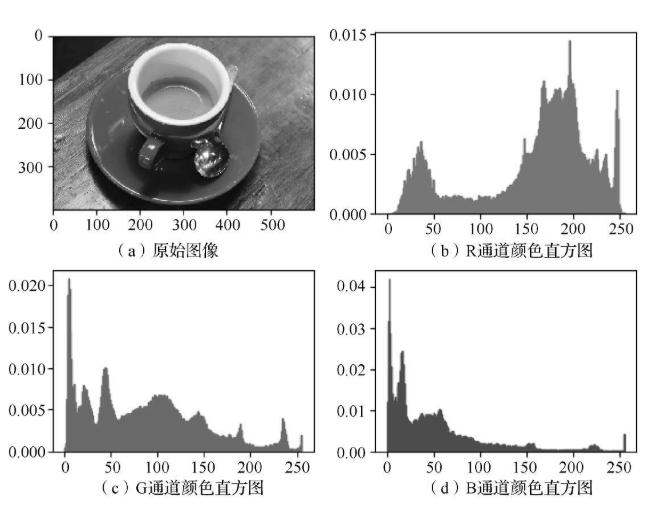• 一般颜色直方图对图像的旋转、小幅平移、小幅缩放等变换不敏感，对图像质量的变化（如增加噪声也不敏感），所以一般颜色直方图法适用于对难以进行语义分割的图像和无需考虑空间位置的图像进行描述。
• 计算机的固有量化机制导致一般颜色直方图法会忽略颜色间的相似性。

#### 2 全局累加直方图

• 当图像中的颜色值不能取遍所有可能的颜色值时，一般颜色直方图中就会出现一些零值。这些零值的出现会影响相似性的度量，进而使计算出的相似度不能准确反映图像之间的颜色分布差异。
• 为了弥补一般颜色直方图的缺陷，再一般颜色直方图的基础上，通过对直方图颜色进行累加，消除零值影响，形成全局累加直方图。

#### 3 主色调直方图

• 在一幅图像中，不同颜色值出现的概率不尽相同，且通常情况下少数几种颜色就能涵盖整幅图像的主色调。
• 基于该思想，主色调直方图会计算出图像中每种颜色出现的频率，选择出现频率最高的几种颜色并将其作为主色调。
• 使用主色调直方图，不会降低颜色直方图匹配的效果，反而会抑制图像非主要成分的噪声，降低噪声对图像匹配的影响。
• 颜色直方图的优点和缺点如下。
• 优点：计算简单，对图像的平移和旋转变换不敏感，能简单描述图像中颜色的全局分布情况。
• 缺点：无法捕捉（即丢失）颜色组成之间的空间位置关系。

### 5.1.2 颜色矩

• 矩是非常重要的统计量，用于表征数据分布的特点。在统计中，一阶矩表示数据的均值，二阶矩表示数据分布的方差，三阶矩表示数据分布的偏移度。
• 图像的颜色矩用于对图像内颜色分布进行表征，是重要的一种全局图像特征表示。
• 数字图像中颜色分布的统计信息主要集中在低阶矩中。
• 图像的颜色矩特征提取时主要瞄准图像颜色矩中的一阶矩、二阶矩、三阶矩，对图像而言，这三种统计特征已经足以表达数字图像的颜色分布。
• 相对于颜色直方图特征提取，颜色矩特征提取的优点是无须对颜色特征进提前量化。
• 一阶矩可以表征该颜色通道的平均响应强度，二阶矩可以表示该颜色通道的响应方差，三阶矩可以表征该颜色通道数据分布的偏移度。
• 针对彩色图像，图像的颜色矩一共有9个分量，每个颜色通道均有3个低阶矩。
• 颜色矩仅使用少数几个矩容易导致过多错误检出，因而其通常和其他的几个特征配合使用。
``````from matplotlib import pyplot as plt
from skimage import data, exposure
import numpy as np
from scipy import stats

# 中文显示工具函数
def set_ch():
from pylab import mpl
mpl.rcParams['font.sans-serif'] = ['FangSong']
mpl.rcParams['axes.unicode_minus'] = False

set_ch()

image = data.coffee()
# 求RGB图像的颜色矩特征，共9维特征
# 定义3*3数组，分别对RGB图像的三个通道求均值、方差、偏移量
features = np.zeros(shape=(3, 3))
for k in range(image.shape):
mu = np.mean(image[:, :, k])  # 求均值
delta = np.std(image[:, :, k])  # 求方差
skew = np.mean(stats.skew(image[:, :, k]))  # 求偏移量
features[0, k] = mu
features[1, k] = delta
features[2, k] = skew
print(features)

``````

### 5.1.3 颜色集

• 颜色集又可以称为颜色索引集，是对颜色直方图的一种近似。
• 颜色集方法的步骤是：第一，将图像从RGB图像空间转换到HSV颜色空间等视觉均衡的颜色空间，并将颜色空间量化为若干个边长均等的小立方体。第二，使用基于颜色的自动分割技术，将图像划分为若干个子区域。第三，使用颜色量化空间中的某个颜色分类索引每个子区域，以将图像表示为一个二进制的颜色索引集。
① 像素矢量表示
② 颜色空间转换
③ 颜色集索引
④ 颜色集表示

### 5.1.4 颜色聚合向量

• 颜色聚合向量是在颜色直方图上做进一步运算，其核心思想就是将属于颜色直方图的每个颜色量化区间的像素分为两部分，如果该颜色量化区间中的某些像素，占据连续区域的面积大于指定阈值，则将该区域内的像素作为聚合像素，否则作为非聚合像素。
• 颜色聚合向量除了包含颜色频率信息外，也包含颜色的部分空间分布信息，因此其可以获得比颜色直方图过更好的效果。颜色聚合向量算法的具体步骤如下。
① 量化
颜色聚合向量算法的第一步与求普通的颜色直方图类似，即对图像进行量化处理。一般采用均匀量化方法，量化的目标是使图像中只保留有限个颜色空间。
② 连通区域划分
针对重新量化后的像素矩阵，根据像素间的连通性把图像划分为若干个连通区域。
③ 判断聚合性
统计每个连通区域中的像素数目，根据设定的阈值判断该区域中的像素的聚合性
④ 聚合向量形成

### 5.1.5 颜色相关图

• 颜色相关图不仅可以显示像素在图像中的占比，也可以反映不同颜色对间的空间位置的相关性。
• 颜色相关图利用颜色对间的相对距离分布来描述空间位置信息。
``````from matplotlib import pyplot as plt
from skimage import data, exposure
import numpy as np
from scipy import stats

# 中文显示工具函数
def set_ch():
from pylab import mpl
mpl.rcParams['font.sans-serif'] = ['FangSong']
mpl.rcParams['axes.unicode_minus'] = False

set_ch()

def isValid(X, Y, point):
"""
判断某个像素是否超出图像空间范围
:param X:
:param Y:
:param point:
:return:
"""
if point < 0 or point >= X:
return False
if point < 0 or point >= Y:
return False
return True

def getNeighbors(X, Y, x, y, dist):
"""
Find pixel neighbors according to various distances
:param X:
:param Y:
:param x:
:param y:
:param dist:
:return:
"""
cn1 = (x + dist, y + dist)
cn2 = (x + dist, y)
cn3 = (x + dist, y - dist)
cn4 = (x, y - dist)
cn5 = (x - dist, y - dist)
cn6 = (x - dist, y)
cn7 = (x - dist, y + dist)
cn8 = (x, y + dist)
points = (cn1, cn2, cn3, cn4, cn5, cn6, cn7, cn8)
Cn = []
for i in points:
if (isValid(X, Y, i)):
Cn.append(i)
return Cn

def corrlogram(image, dist):
XX, YY, tt = image.shape
cgram = np.zeros((256, 256), dtype=np.int)
for x in range(XX):
for y in range(YY):
for t in range(tt):
color_i = image[x, y, t]
neighbors_i = getNeighbors(XX, YY, x, y, dist)
for j in neighbors_i:
j0 = j
j1 = j
color_j = image[j0, j1, t]
cgram[color_i, color_j] = cgram[color_i, color_j] + 1
return cgram

img = data.coffee()
dist = 4
cgram = corrlogram(img, dist)
plt.imshow(cgram)
plt.show()

``````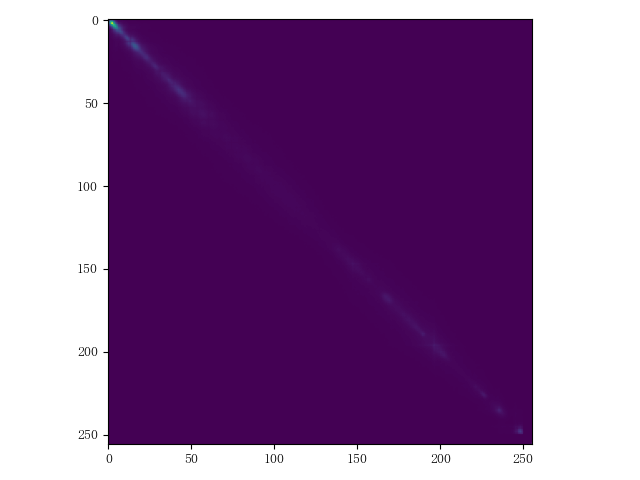## 5.2 图像纹理特征提取

• 纹理是一种反映图像中同质现象的视觉特征，它体现了物体表面的具有重复性或周期性变化的表面结构组织排列属性。纹理有三大特点：重复性、周期性、同质性。
① 重复性
图像可以看作是某种局部元素在全局区域的不断重复出现。
② 周期性
图像中元素并非随机出现，而是按照一定的周期性重复出现。
③ 同质性
重复出现的元素在结构和尺寸上大致相同。
• 纹理是某种局部序列不断重复、非随机排列、在结构和尺寸上大致相同的统一体。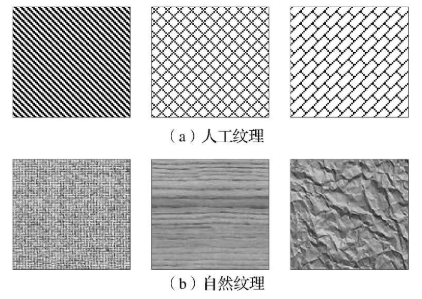• 不同于灰度、颜色等图像特征，纹理特征通过像素及其周边空间域像素的灰度分布进行描述，也就是局部纹理信息。局部纹理的反复排布呈现出的重复性，就是所谓的全局纹理信息。
• 纹理信息在表现全局特征性质的同时，也体现了图像或图像所在区域对应景物的表面特性。
• 纹理特征只是对物体表面特性进行描述，并不能反映物体的本质属性，即图像高层语义信息。
• 纹理特征提取过程是通过一定的图像处理技术抽取出纹理特征，从而获得纹理的定量或定性描述的过程。纹理特征提取的基本过程如下。
① 纹理基元建模
从像素出发，找出纹理基元（即纹理图像中辨识能力比较强的特征），并根据纹理基元的排列信息，建立起纹理基元模型。
② 整体纹理模型构建
利用纹理基元模型对纹理图像进行特征提取，以支持对图像的进一步分割、分类以及辨识，形成图像整体纹理模型。
• 常见的纹理特征提取方法可以分为如下4类。
① 统计分析法
统计分析法又称基于统计纹理特征的检测方法，该方法假设纹理图像在空间灰度分布上，存在某种重复性，通过对纹理图像的灰度空间分布进行计算，从而得到纹理特征，主要包括灰度直方图法、灰度共生矩阵法、灰度行程长度法、灰度差分统计、交叉对角矩阵、自相关函数法等。该类方法在木纹、沙地、草地之类的图像分析上很有效。其主要优势是：方法简单，易于实现，典型的代表方法是灰度共生矩阵法，被认为是比较有效的纹理分析方法。
② 结构分析法
结构分析法认为纹理基元之间存在某种结构规则关系，该类方法首先对图像中的纹理基元进行分离，然后基于纹理基元的特征和空间排列规则对纹理进行表征，主要包括偏心度、面积、方向等特征，其主要目标是通过图像结构特征和排列规则的描述，得到纹理特征的描述，此类算法主要适用于已知纹理基元的情况，对砖墙、纤维等纹理基元和排列规则都比较明显的图像有效。
③ 模型分析法
模型分析法基于像素及其邻域像素之间的关系建立模型，根据不同模型提取不同特征量，进行参数估计。典型的模型分析法包括自然回归法、马尔可夫条件随机场法以及分形法等。
④ 频谱分析法
频谱分析法又称为信号处理法和滤波方法。该方法将纹理图像从空间域变换到频域，然后通过计算峰值处的面积、峰值与原点的距离平方、峰值处的相位、两个峰值间的相角差等，获得在空间域不易获得的纹理特征，如周期、功率谱信息等，典型的频谱分析法有二维傅里叶（变换）滤波方法，Gabor（变换）滤波变换和小波方法等。

### 5.2.1 统计纹理分析方法

• 统计纹理分析方法是较常用的纹理特征描述分析方法，通过统计图像的空间频率、边界频率以及空间灰度依赖关系等对纹理进行描述。
• 细致的纹理有较高的频率，例如，布匹的纹理是非常细致的纹理，其纹理基元较小，出现频率较高。
• 粗糙的纹理结构具有较低的空间频率，如大理石纹理一般较粗糙，具有较大的纹理基元，出现频率较低。
• 纹理图像的空间频率可以作为纹理描述的一种方式。
• 边界频率是另外一种基于统计的纹理图像描述方法，边界频率越高，表明纹理越精细。
• 空间灰度依赖关系方法通过描述纹理结构之间的空间依赖关系描述纹理。
• 常用的统计纹理分析方法有自相关函数、边界频率、灰度共生矩阵等。
• 统计纹理分析方法并不刻意精确描述纹理的结构。
• 从统计学的角度看，纹理图像是一些复杂的模式，通常通过获得的统计特征集描述这些模式。
• 灰度共生矩阵法也称联合概率矩阵法。该方法基于图像中的灰度结构重复出现的概率，对图像纹理特征进行描述。该方法的本质是使用条件概率表征纹理特征，通过对空间上，具有某种位置关系的一对像素成对出现的概率进行统计，得到灰度共生矩阵，然后从灰度共生矩阵中提取有意义的统计特征对纹理进行描述。
``````from matplotlib import pyplot as plt
from skimage.feature import greycomatrix, greycoprops
from skimage import data
import numpy as np

# 中文显示工具函数
def set_ch():
from pylab import mpl
mpl.rcParams['font.sans-serif'] = ['FangSong']
mpl.rcParams['axes.unicode_minus'] = False

set_ch()
PATCH_SIZE = 21
image = data.camera()
# 选择图像中的草地区域
grass_locations = [(474, 291), (440, 433), (466, 18), (462, 236)]
grass_patches = []
for loc in grass_locations:
grass_patches.append(image[loc:loc + PATCH_SIZE, loc:loc + PATCH_SIZE])

# 选择图像中的天空区域
sky_locations = [(54, 48), (21, 233), (90, 380), (195, 330)]
sky_patches = []
for loc in sky_locations:
sky_patches.append(image[loc:loc + PATCH_SIZE, loc:loc + PATCH_SIZE])
# 计算每个块中灰度共生矩阵属性
xs = []
ys = []
for patch in (grass_patches + sky_patches):
glcm = greycomatrix(patch, , , 256, symmetric=True, normed=True)
xs.append(greycoprops(glcm, 'dissimilarity')[0, 0])
ys.append(greycoprops(glcm, 'correlation')[0, 0])
# 创建绘图
fig = plt.figure(figsize=(8, 8))
# 展现原始图像，以及图像块的位置
ax.imshow(image, cmap=plt.cm.gray, interpolation='nearest', vmin=0, vmax=255)
for (y, x) in grass_locations:
ax.plot(x + PATCH_SIZE / 2, y + PATCH_SIZE / 2, 'gs')
for (y, x) in sky_locations:
ax.plot(x + PATCH_SIZE / 2, y + PATCH_SIZE, 'bs')
ax.set_xlabel('原始图像')
ax.set_xticks([])
ax.set_yticks([])
ax.axis('image')
# 对于每个块，plot(dissimilarity,correlation)
ax.plot(xs[:len(grass_patches)], ys[:len(grass_patches)], 'go', label='Grass')
ax.plot(xs[:len(sky_patches)], ys[:len(sky_patches)], 'bo', label='Sky')
ax.set_xlabel('灰度共生矩阵相似性')
ax.set_ylabel('灰度共生矩阵相关度')
ax.legend()
# 展示图像
for i, patch in enumerate(grass_patches):
ax = fig.add_subplot(3, len(grass_patches), len(grass_patches) * 1 + i + 1)
ax.imshow(patch, cmap=plt.cm.gray, interpolation='nearest', vmin=0, vmax=255)
ax.set_xlabel('Grass %d' % (i + 1))
for i, patch in enumerate(sky_patches):
ax = fig.add_subplot(3, len(sky_patches), len(sky_patches) * 2 + i + 1)
ax.imshow(patch, cmap=plt.cm.gray, interpolation='nearest', vmin=0, vmax=255)
ax.set_xlabel('Sky %d' % (i + 1))
# 展示图像块并显示
fig.suptitle('Grey level co-occurrence matrix features', fontsize=14)
plt.show()

``````
• 基于灰度共生矩阵的纹理描述方法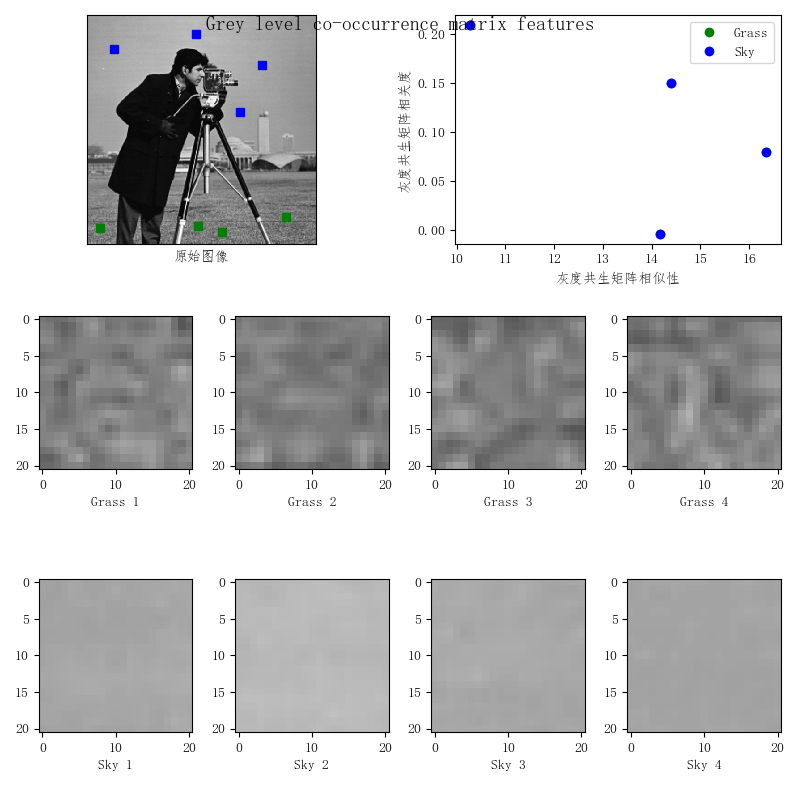### 5.2.2 Laws纹理能量测量法

• Laws纹理能量测量法是一种典型的一阶纹理分析方法，在纹理分析领域有一定影响。
• Laws纹理能力测量的基本思想是创建两个窗口：一个是微窗口，可为3×3、5×5或7×7，常取5×5测量以像元为中心的小区域的灰度的不规则性，以形成属性，称为微窗口滤波；另一个是宏窗口，为15×15或32×32，用来在更大的区域上求属性的一阶统计量（常为均值和标准偏差），称为能量变换。
• 整个纹理分析过程为：`f(x,y)`→微窗口滤波→`F(x,y)`→能量转换→`E(x,y)`→分类

### 5.2.3 Gabor变换

• 大量心理和生理学研究发现，在人类的低级视觉中，输入信号被一系列具有不同频率和方位的线性空间滤波器分解成一组频率和方位通道，Gabor分解可以很好的描述这一信号分解过程。
• Gabor变换具有两个重要的特性。一是其良好的空间域与频域局部化性质。二是无论从空间域的起伏特性上，方位选择特性上，空间域与频域选择上，还是从正交相位的关系上，二维Gabor基函数具有与大多数哺乳动物的视觉表皮简单细胞的二维感知域模型相似的性质。
• 可以借鉴人类处理信号的特性，用包含多个Gabor滤波器的滤波器组，对图像进行不同中心频率和方位的滤波处理，从而提取不同频率成分和不同方位的特征，作为目标的非参数化特征，研究其不同分辨率目标的特征与图像分辨率的关系。
• Gabor滤波器滤波过程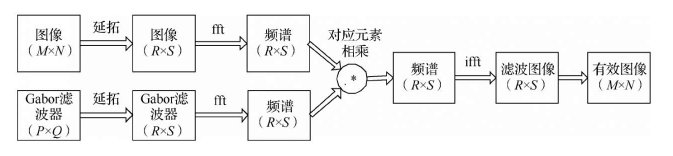### 5.2.4 局部二值模式

• 局部二值模式（Local Binary Pattern，LBP）的基本思想是将中心像素点的灰度值作为阈值，将其邻域内的像素点灰度值与阈值进行比较，从而得到二进制编码用以表述局部纹理特征。
• LBP表示方法不易受图像整体灰度线性变化的影响，当图像的灰度值发生线性均匀变化时，其LBP特征编码是不变的。
• LBP特征计算简单，表征能力强，在纹理特征描述上具有较好的效果。
• 基本LBP算子：3*3的矩形块，有一个中心像素和8个邻域像素，分别对应9个灰度值。
• 特征值：以中心像素的灰度值为阈值，将其邻域的8个灰度值与阈值比较，大于中心灰度值的像素用1表示，反之用0表示。然后顺时针方向读出8个二进制值。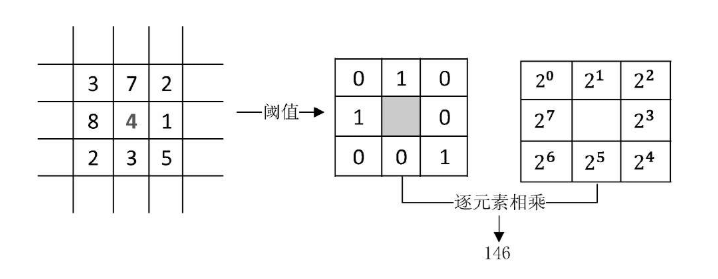• 经阈值化后的二值矩阵，可以看出一个二值纹理模式，用来刻画邻域内，像素点的灰度相对中心点的变化。
• 因为人类视觉系统对纹理的感知与平均灰度（亮度无关），而局部二值模式方法注重像素灰度的变化，符合人类视觉对纹理感知的特点。
``````import skimage.feature
import skimage.segmentation
import skimage.data
import matplotlib.pyplot as plt

# 中文显示工具函数
def set_ch():
from pylab import mpl
mpl.rcParams['font.sans-serif'] = ['FangSong']
mpl.rcParams['axes.unicode_minus'] = False

set_ch()
img = skimage.data.coffee()
for colour_channel in (0, 1, 2):
img[:, :, colour_channel] = skimage.feature.local_binary_pattern(img[:, :, colour_channel], 8, 1.0, method='var')
plt.imshow(img)
plt.show()

``````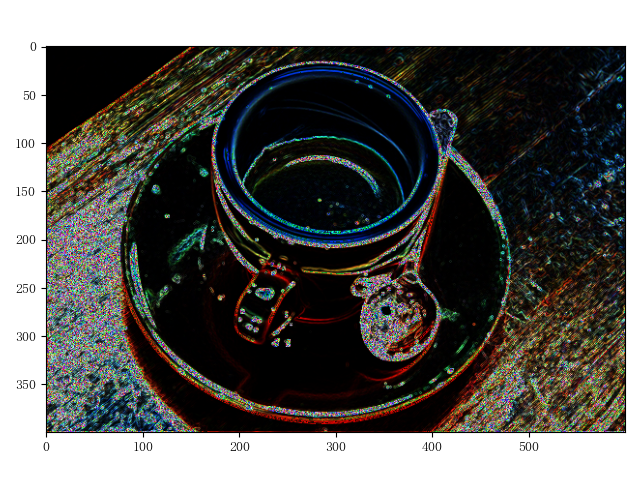## 5.3 图像形状特征提取

• 形状和区域特征是图像中的另一类重要特征。
• 不同于颜色、纹理等底层特征，对形状特征的描述必须以对图像中的物体或区域对象的分割为前提。
• 形状特征的表示方法可以分为两类：一是基于轮廓特征，典型方法是傅里叶描述符方法。二是基于区域特征，典型方法是形状无关矩法。
• 轮廓特征中只用到物体的边界，而区域特征需要考虑整个形状区域。

### 5.3.1 简单形状特征

• 矩形度。反应物体对外接矩形的充满度，用物体的面积与其最小外接矩形的面积之比描述。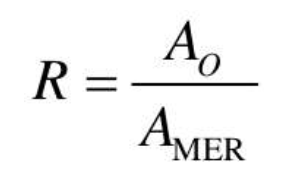• 球状性。球状性既可以描述二维目标，也可描述三维目标。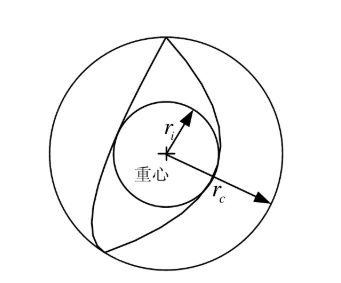• 圆形性。目标圆形性是指用目标区域R的所有边界点定义的特征量。

### 5.3.2 傅里叶描述符

• 傅里叶描述符是用于单封闭曲线形状特征描述的常用工具。
• 傅里叶描述符将待描述目标曲线看组一维数值序列，使用傅里叶变换对该序列进行转换，得到描述该曲线的一系列傅里叶系数。
• 傅里叶描述符具有计算简单、描述清晰等优点。相较于其他复杂的描述方法，傅里叶描述符更加直观，易于理解。
• 傅里叶描述方法一般分为两步：首先，定义轮廓线的表示，把坐标的序列点看作复数，即`s(k)`=`x(k)`+`jy(k)`，x轴作为实轴，y轴作为虚轴，边界的性质不变。这种表示方法的优点是将一个二维边缘描述问题，简化为一个一维序列描述问题。其次，对一维序列s(k)进行傅里叶变换，并求得其傅里叶系数。

### 5.3.3 形状无关矩

• 由于图像区域的某些矩对于平移、旋转、尺度等几何变换具有一些不变的特性，使得矩的表示方法在物体的分类与识别方面具有重要的意义。

## 5.4 图像边缘特征提取

• 图像边缘具有方向和幅度两个主要成分。沿边缘方向移动，像素的灰度值变化速率较为平缓，而沿垂直于边缘的方向移动，像素的灰度值变化率较为剧烈。这种剧烈的变化或者呈阶跃状（Step Edge），或呈屋顶状（Proof Edge），分为称为阶跃状边缘和屋顶状边缘。根据边缘的性质，一般用一阶或二阶导数对其进行描述和检测。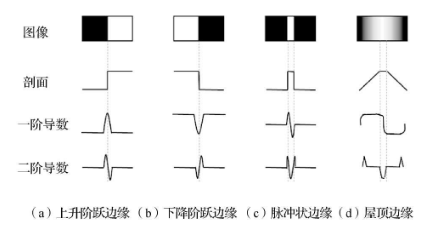• 图像中的边缘可以通过对他们求导数确定，而导数可利用微分算子计算。对于数字图像处理而言，通常利用差分近似微分。
• 图像边缘检测的基本步骤如下。
① 滤波
边缘检测主要基于导数计算，但易受噪声影响，滤波操作的主要目的是降低噪声的干扰，但滤波在降低噪声的同时，也会损失边缘强度。
② 增强
增强算法将局部邻域中灰度值有显著变化的点突出显示，一般可通过计算梯度幅值完成。
③ 检测
有些图像中梯度幅值较大的点并不是边缘点，需要对其进行进一步筛选，最简单的检测方法是设定梯度幅值阈值。
④ 定位
定位即精确确定边缘的位置。
• 传统边缘检测的流程。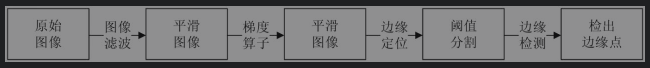### 5.4.1 梯度边缘检测

• 梯度的方向（由梯度矢量的幅角表示）是函数f(x,y)增加最快的方向，定义为`ϕ(x,y)`=`arctan(Gy/Gx)`，从梯度原理出发，已经发展了很多边缘检测算子。

### 5.4.2 一阶边缘检测算子

#### 1 罗伯特算子

• 罗伯特边缘检测算子，用对角线上相邻像素之差代替梯度寻找边缘。
• 罗伯特边缘检测的步骤：
（1）用两个模板分别对图像进行运算得到`Rxf``Ryf`，并计算`|G(i,j)|`=`|Gx|`+`|Gy|`
（2）判断该相加结果是否大于某个阈值。如果满足条件，则将其作为结果图像中对应模板(i,j)位置的像素值；如果不满足条件，则结合结果图像中对应模板`(i,j)`位置的像素赋0值。
``````import matplotlib.pyplot as plt
from skimage.data import camera
from skimage.filters import roberts

# 中文显示工具函数
def set_ch():
from pylab import mpl
mpl.rcParams['font.sans-serif'] = ['FangSong']
mpl.rcParams['axes.unicode_minus'] = False

set_ch()
image = camera()
edge_roberts = roberts(image),
fig, ax = plt.subplots(ncols=2, sharex=True, sharey=True, figsize=(8, 4))
ax.imshow(image, cmap='gray')
ax.set_title('原始图像')

ax.imshow(edge_roberts, cmap='gray')
ax.set_title('Roberts 边缘检测')
for a in ax:
a.axis('off')
plt.tight_layout()
plt.show()

``````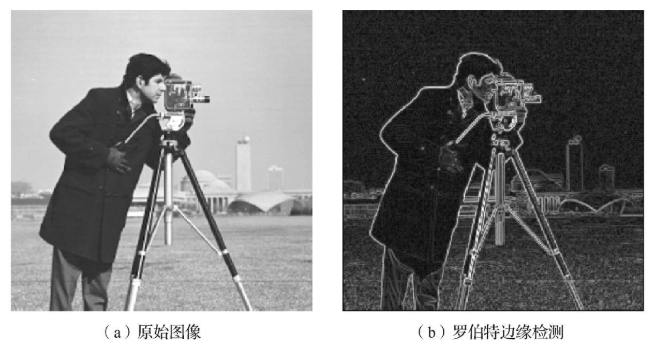#### 2 索贝尔算子

• 索贝尔边缘检测的步骤：
（1）用两个模板分别对图像进行计算，得出`|G(i,j)|`=`Gx`+`Gy`
（2）判别该相加结果是否大于或等于某个阈值，如果满足条件，则将其作为结果图像中对应模板`(i,j)`位置的像素值；如果不满足条件，则给结果图像中对应模板`(i,j)`位置的像素赋0值。
``````import matplotlib.pyplot as plt
from skimage.data import camera
from skimage.filters import sobel, sobel_v, sobel_h

# 中文显示工具函数
def set_ch():
from pylab import mpl
mpl.rcParams['font.sans-serif'] = ['FangSong']
mpl.rcParams['axes.unicode_minus'] = False

set_ch()
image = camera()
edge_sobel = sobel(image)
edge_sobel_v = sobel_v(image)
edge_sobel_h = sobel_h(image)
fig, ax = plt.subplots(ncols=2, nrows=2, sharex=True, sharey=True, figsize=(8, 4))

ax[0, 0].imshow(image, cmap=plt.cm.gray)
ax[0, 0].set_title('原始图像')

ax[0, 1].imshow(edge_sobel, cmap=plt.cm.gray)
ax[0, 1].set_title('Sobel 边缘检测')

ax[1, 0].imshow(edge_sobel_v, cmap=plt.cm.gray)
ax[1, 0].set_title('Sobel 垂直边缘检测')

ax[1, 1].imshow(edge_sobel_h, cmap=plt.cm.gray)
ax[1, 1].set_title('Sobel 水平边缘检测')

for a in ax:
for j in a:
j.axis('off')
plt.tight_layout()
plt.show()

``````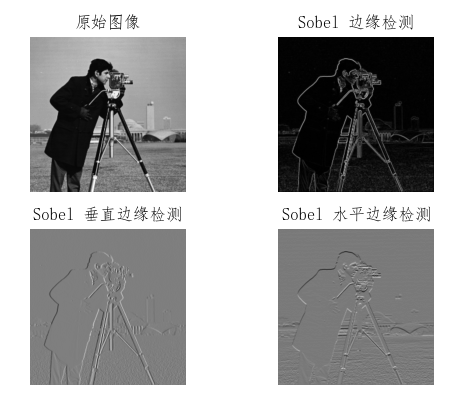• 索贝尔边缘检测算子在较好的获得边缘性效果的同时，对噪声具有一定的平滑作用，减少了噪声的敏感性。但索贝尔边缘检测的边缘比较粗，会检测出一些伪边缘，边缘检测精度较低。

#### 3 Prewitt算子

• Prewitt算子在方向和方向的梯度幅值上的形式，与索贝尔算子的形式完全相同，只是系数均为1，对应的3*3模板为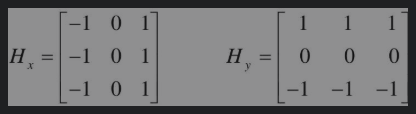• Prewitt算子的计算比索贝尔算子更简单，但在噪声抑制方面，索贝尔算子比Prewitt算子略胜一筹。

• 梯度算子对噪声有一定的敏感性，所以适用于图像边缘灰度值比较尖锐，且图像中噪声比较小的情况

### 5.4.3 二阶边缘检测算子

• 在利用一阶导数的边缘检测算子进行边缘检测时，有时会出现因检测到的边缘点过多，而导致边缘线过粗的情况。
• 通过去除一阶导数中的非局部最大值，就可以检测出更细的边缘，而一阶导数的局部最大值对应二阶导数的零交叉点。所以，通过找图像的二阶导数的零交叉点，就能找到精确的边缘点。

#### 1 拉普拉斯（Laplace）算子

• 对阶跃状边缘，其二阶导数在边缘点出现过零交叉，即边缘点两旁的二阶导数取异号，据此可通过二阶导数检测边缘点。
• Laplace算子的边缘检测
``````import matplotlib.pyplot as plt
from skimage.data import camera, coffee
from skimage.filters import laplace

# 中文显示工具函数
def set_ch():
from pylab import mpl
mpl.rcParams['font.sans-serif'] = ['FangSong']
mpl.rcParams['axes.unicode_minus'] = False

set_ch()
image_camera = camera()
edge_laplace_camera = laplace(image_camera)
image_coffee = coffee()
edge_laplace_coffee = laplace(image_coffee)
fig, ax = plt.subplots(ncols=2, nrows=2, sharex=True, sharey=True, figsize=(8, 6))

ax[0, 0].imshow(image_camera, cmap=plt.cm.gray)
ax[0, 0].set_title('原始图像')

ax[0, 1].imshow(edge_laplace_camera, cmap=plt.cm.gray)
ax[0, 1].set_title('Laplace 边缘检测')

ax[1, 0].imshow(image_coffee, cmap=plt.cm.gray)
ax[1, 0].set_title('原始图像')

ax[1, 1].imshow(edge_laplace_coffee, cmap=plt.cm.gray)
ax[1, 1].set_title('Laplace 边缘检测')

for a in ax:
for j in a:
j.axis('off')
plt.tight_layout()
plt.show()

``````
• 边缘检测算子模板的基本特征是中心位置的系数为正，其余位置的系数为负，且模板的系数之和为0.
• 它的使用方法是用图中的两个点阵之一作为卷积核，与原图像进行卷积运算即可。
• Lapace检测模板的特点是各向同性，对孤立点及线端的检测效果好，Laplace算子的缺点是会出现边缘方向信息丢失，对噪声敏感，整体检测效果不如梯度算子，且与索贝尔算子相比，对图像进行处理时，Laplace算子能使噪声成分得到加强，对噪声更敏感。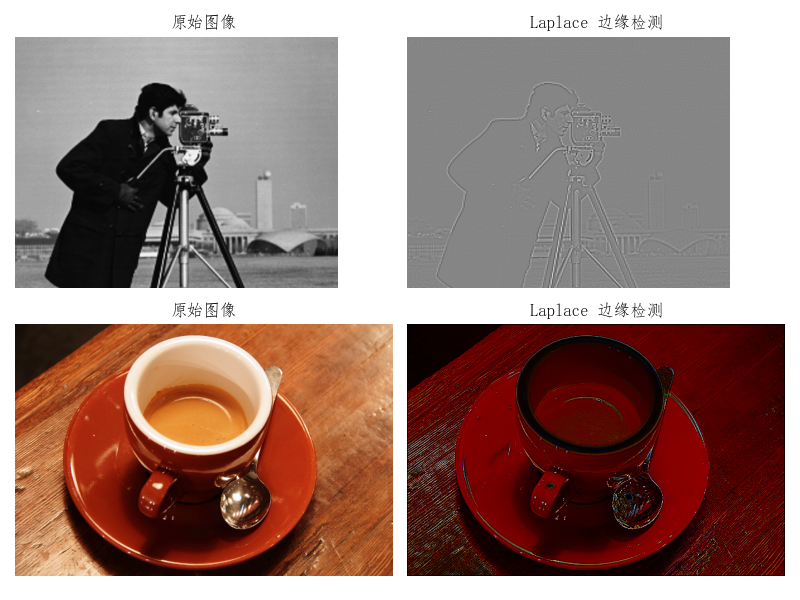#### 2 LoG边缘检测算子

• 实际应用中，由于噪声的影响，对噪声敏感的边缘检测点检测算法（如拉普拉斯算子法）可能会把噪声当边缘检测点检测出来，而真正的边缘点会被噪声淹没而未检测出。
• LoG算子基于Laplace算子和Gauss算子，也称拉普拉斯-高斯边缘检测算子。
• 由于平滑会导致边缘的延展，因此在边缘检测时，仅考虑那些具有局部最大值的点为边缘点。
``````import matplotlib.pyplot as plt
from skimage.data import camera, coffee
from skimage.filters import laplace, gaussian

# 中文显示工具函数
def set_ch():
from pylab import mpl
mpl.rcParams['font.sans-serif'] = ['FangSong']
mpl.rcParams['axes.unicode_minus'] = False

set_ch()
image = camera()
edge_laplace = laplace(image)
gaussian_image = gaussian(image)
edge_LoG = laplace(gaussian_image)

fig, ax = plt.subplots(ncols=2, nrows=2, sharex=True, sharey=True, figsize=(8, 6))

ax[0, 0].imshow(image, cmap=plt.cm.gray)
ax[0, 0].set_title('原始图像')

ax[0, 1].imshow(edge_laplace, cmap=plt.cm.gray)
ax[0, 1].set_title('Laplace 边缘检测')

ax[1, 0].imshow(gaussian_image, cmap=plt.cm.gray)
ax[1, 0].set_title('高斯平滑后的图像')

ax[1, 1].imshow(edge_LoG, cmap=plt.cm.gray)
ax[1, 1].set_title('LoG 边缘检测')

for a in ax:
for j in a:
j.axis('off')
plt.tight_layout()
plt.show()

``````
• 基于LoG的边缘检测效果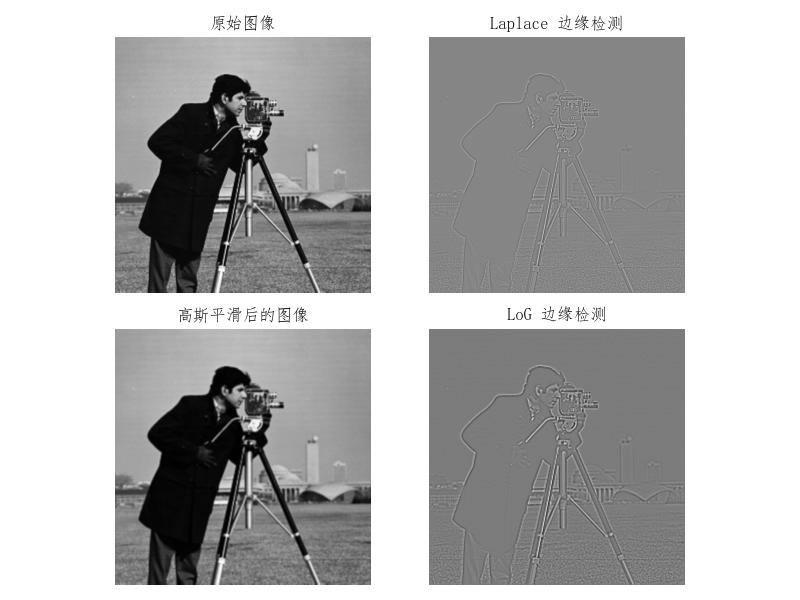## 5.5 图像点特征提取

• 如果图像中一个非常小的区域的灰度值，与其邻域值相比有明显的差异，则称这个非常小的区域为图像点（一般意义上的孤立像素点）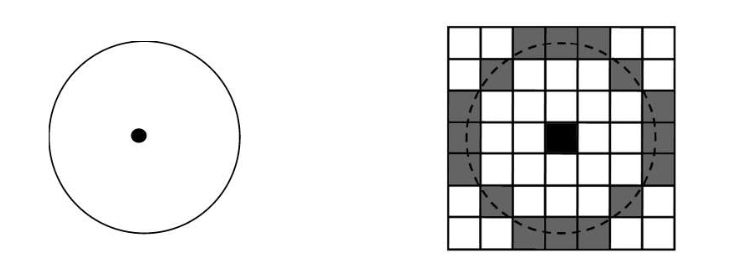• 目前对图像点特征提取的技术有多种，其中研究最多、应用最广的是角点检测算法。
• 从直观可视的角度出发，两条直线相交的顶点可看作是角点；物体的几个平面的相交处也可以看作角点。从图像特征的角度出发，图像中周围灰度变化较剧烈的点可看作是角点；图像边界上曲率足够高的点也可以看作是角点。
• 常见的角点类型。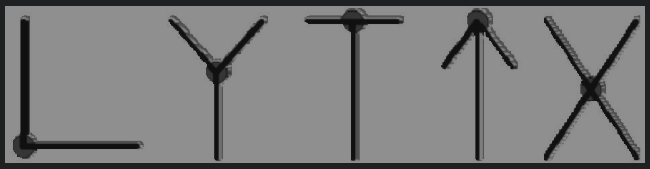• 角点的检测方法有很多种，其检测原理也多种多样，但这些方法概括起来大体可分为3类：一是基于模板的角点检测算法；二是基于边缘的角点检测算法；三是基于图像灰度变化的角点检测算法。其中，基于图像灰度变化的角点检测算法应用最广泛。
• SUSAN角点检测算法选用圆形模板，将位于圆形窗口模板中心等待检测的像素点称为核心点。核心点的邻域被划分为两个区域：亮度值相似于核心点亮度的区域即核值相似区和亮度值不相似于核心点亮度的区域。
• SUSAN算法通过核值相似区的大小判别图像角点，并实现图像中角点特征的检测和提取。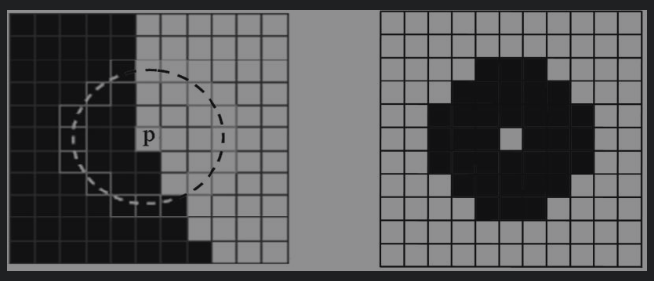• SUSAN算子实现代码
``````import matplotlib.pyplot as plt
from skimage.data import camera
import numpy as np

# 中文显示工具函数
def set_ch():
from pylab import mpl
mpl.rcParams['font.sans-serif'] = ['FangSong']
mpl.rcParams['axes.unicode_minus'] = False

def susan_corner_detection(img):
img = img.astype(np.float64)
g = 37 / 2
output = np.zeros(img.shape)
for i in range(3, img.shape - 3):
for j in range(3, img.shape - 3):
ir = np.array(img[i - 3:i + 4, j - 3:j + 4])
ir0 = img[i, j]
a = np.sum(np.exp(-((ir - ir0) / 10) ** 6))
if a <= g:
a = g - a
else:
a = 0
output[i, j] = a
return output

set_ch()
image = camera()
out = susan_corner_detection(image)
plt.imshow(out, cmap='gray')
plt.show()

``````
• 基于SUSAN算子的角点检测响应图像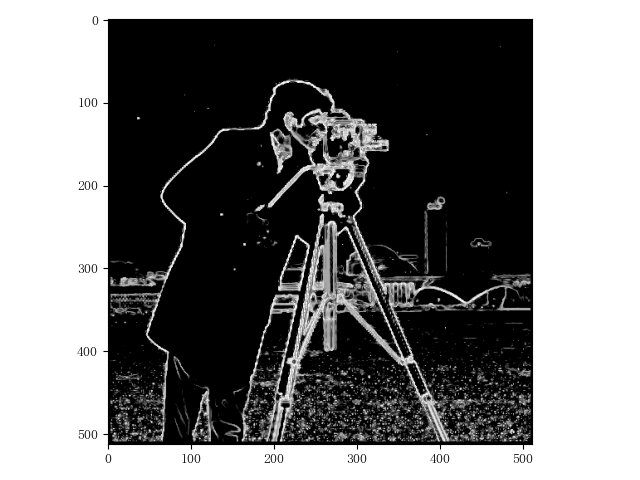## 5.6 小结

• 本章首先对图像特征提取进行了简单介绍，其次主要介绍了全局特征提取技术，如颜色、纹理、形状特征提取，最后对局部特征提取技术（如边缘检测、角点检测）进行了简单介绍。